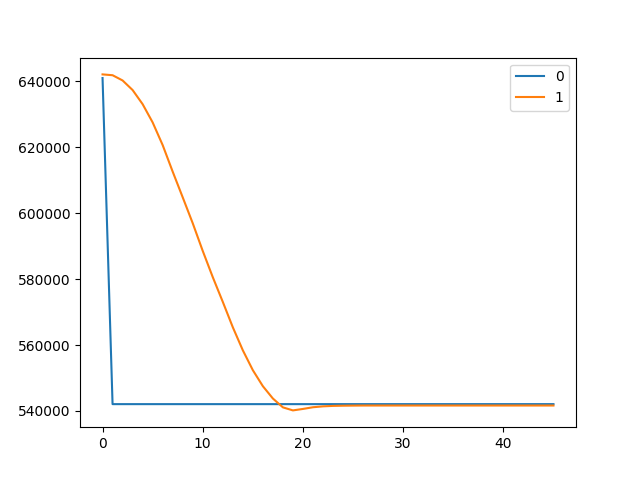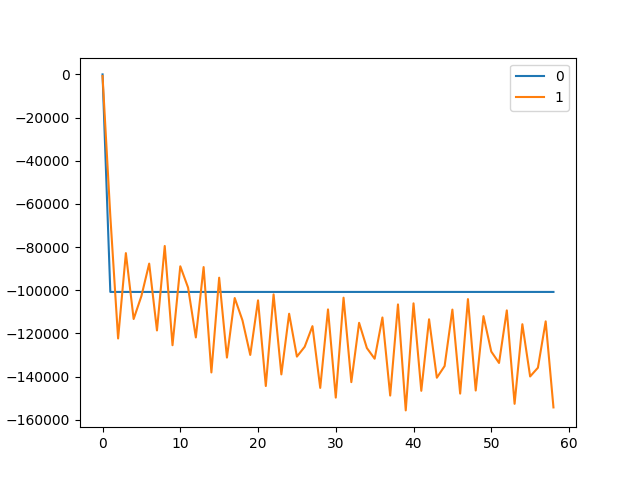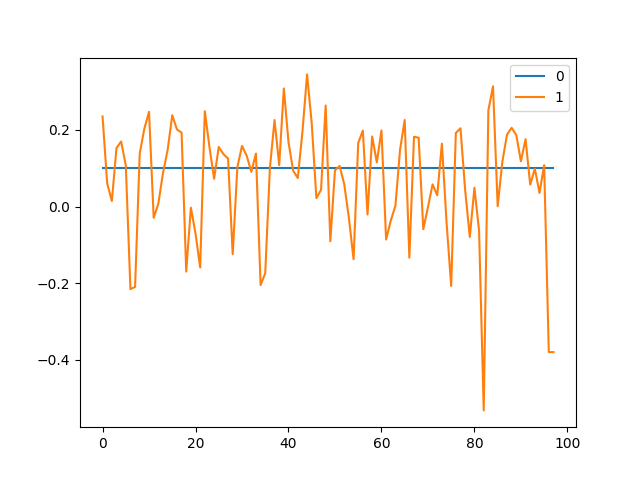# Troubles with small BLDC motor and ODrive

Hi everyone,

I’m working with a Nanotec BLDC motor 416.67 kv and I can’t seem to get it tuned correctly. I’ve tried following the tuning guidelines no luck. The calibration causes no errors and the encoders AMT103-V 2048 PPR I’m using seem to work as well.

Here are my configs.
axis
startup_motor_calibration = False (bool)
startup_encoder_index_search = False (bool)
startup_encoder_offset_calibration = False (bool)
startup_closed_loop_control = False (bool)
startup_sensorless_control = False (bool)
enable_step_dir = False (bool)
counts_per_step = 2.0 (float)
step_gpio_pin = 1 (int)
dir_gpio_pin = 2 (int)
ramp_up_time = 0.4000000059604645 (float)
ramp_up_distance = 12.566370964050293 (float)
spin_up_current = 10.0 (float)
spin_up_acceleration = 400.0 (float)
spin_up_target_vel = 400.0 (float)

controller
control_mode = 3 (int)
pos_gain = 7.0 (float)
vel_gain = 1.0000000116860974e-07 (float)
vel_integrator_gain = 0.0 (float)
vel_limit = 80000000.0 (float)
vel_limit_tolerance = 1.2000000476837158 (float)
vel_ramp_rate = 1.0 (float)
setpoints_in_cpr = False (bool)

motor
pre_calibrated = True (bool)
pole_pairs = 2 (int)
calibration_current = 0.949999988079071 (float)
resistance_calib_max_voltage = 8.0 (float)
phase_inductance = 0.0010457956232130527 (float)
phase_resistance = 2.7222201824188232 (float)
direction = 1 (int)
motor_type = 0 (int)
current_lim = 2.700000047683716 (float)
requested_current_range = 2.700000047683716 (float)
current_control_bandwidth = 10.0 (float)

encoder
mode = 0 (int)
use_index = True (bool)
pre_calibrated = True (bool)
idx_search_speed = 10.0 (float)
zero_count_on_find_idx = True (bool)
cpr = 8192 (int)
offset = 16372 (int)
offset_float = 1.0242187976837158 (float)
bandwidth = 10.0 (float)
calib_range = 0.019999999552965164 (float)

calibration values
current_meas_phB = -0.13242359459400177 (float)
current_meas_phC = 0.017752788960933685 (float)
DC_calib_phB = -0.04886918514966965 (float)
DC_calib_phC = -0.11841728538274765 (float)
phase_current_rev_gain = 0.012500000186264515 (float)
current_control:
p_gain = 0.010457956232130527 (float)
i_gain = 27.22220230102539 (float)
v_current_control_integral_d = -0.05697176977992058 (float)
v_current_control_integral_q = -0.03862487152218819 (float)
Ibus = 0.00032590687624178827 (float)
final_v_alpha = 0.03676741197705269 (float)
final_v_beta = -0.057533249258995056 (float)
Iq_setpoint = 0.0013523530215024948 (float)
Iq_measured = -0.1383826732635498 (float)
max_allowed_current = 30.375 (float)
overcurrent_trip_level = 33.75 (float)

Some plots I have.I can only seem to post one picture, but I cannot follow a sinusoid. of a similar amplitude. If I set to circular position, there are also issues.

Any help is much appreciated!

Set your velocity and current bandwidths higher. Like 1000 not 10.

It’s very unstable with higher values. I can post some plots.

Here’s what happens when I increase the velocity and current bandwidths.I think I’ve narrowed it down to a current control issue. Here I’ve set my current setpoint to 0.1 and I get very high variability in current.ODrive will have a hard time controlling such a low current motor. The lowest gain value possible is intended for 20A motors. Also, does 2.722 ohms sound right for the phase resistance? That’s high, getting into the “gimbal motor” range.

Do you think replacing the shunt resistor is worth exploring? In this topic it seems like a similar issue.

I am using smaller motors and the vel_gain is around .0001 anything over that and the motor vibrates. Hope this helps.